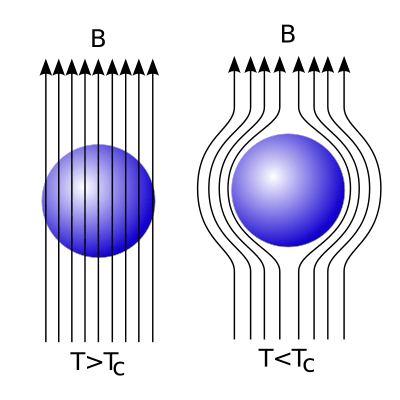# London equationsAs a material drops below its superconducting critical temperature, magnetic fields within the material are expelled via the Meissner effect. The London equations give a quantitative explanation of this effect.

The London equations, developed by brothers Fritz and Heinz London in 1935, relate current to electromagnetic fields in and around a superconductor. Arguably the simplest meaningful description of superconducting phenomena, they form the genesis of almost any modern introductory text on the subject. A major triumph of the equations is their ability to explain the Meissner effect, wherein a material exponentially expels all internal magnetic fields as it crosses the superconducting threshold.

## Formulations

There are two London equations when expressed in terms of measurable fields:Hereis the superconducting current density, E and B are respectively the electric and magnetic fields within the superconductor,is the charge of an electron & proton,is electron mass, andis a phenomenological constant loosely associated with a number density of superconducting carriers. Throughout this article SI units are employed.

On the other hand, if one is willing to abstract away slightly, both the expressions above can more neatly be written in terms of a single "London Equation" in terms of the vector potential A:The last equation suffers from only the disadvantage that it is not gauge invariant, but is true only in the Coulomb gauge, where the divergence of A is zero. This equation holds for magnetic fields that vary slowly in space.

## London penetration depth

If the second of London's equations is manipulated by applying Ampere's law,,

then the result is the differential equationThus, the London equations imply a characteristic length scale,, over which external magnetic fields are exponentially suppressed. This value is the London penetration depth.

For an example, consider a superconductor within free space where the magnetic field outside the superconductor is a constant value pointed parallel to the superconducting boundary plane in the z direction. If x leads perpendicular to the boundary then the solution inside the superconductor may be shown to beFrom here the physical meaning of the London penetration depth can perhaps most easily be discerned.

## Rationale for the London equations

### Original arguments

While it is important to note that the above equations cannot be formally derived, the Londons did follow a certain intuitive logic in the formulation of their theory. Substances across a stunningly wide range of composition behave roughly according to Ohm's law, which states that current is proportional to electric field. However, such a linear relationship is impossible in a superconductor for, almost by definition, the electrons in a superconductor flow with no resistance whatsoever. To this end, the London brothers imagined electrons as if they were free electrons under the influence of a uniform external electric field. According to the Lorentz force lawthese electrons should encounter a uniform force, and thus they should in fact accelerate uniformly. This is precisely what the first London equation states.

To obtain the second equation, take the curl of the first London equation and apply Faraday's law,,

to obtainAs it currently stands, this equation permits both constant and exponentially decaying solutions. The Londons recognized from the Meissner effect that constant nonzero solutions were nonphysical, and thus postulated that not only was the time derivative of the above expression equal to zero, but also that the expression in the parentheses must be identically zero. This results in the second London equation.

### Canonical momentum arguments

It is also possible to justify the London equations by other means. Current density is defined according to the equationTaking this expression from a classical description to a quantum mechanical one, we must replace values j and v by the expectation values of their operators. The velocity operatoris defined by dividing the gauge-invariant, kinematic momentum operator by the particle mass m. We may then make this replacement in the equation above. However, an important assumption from the microscopic theory of superconductivity is that the superconducting state of a system is the ground state, and according to a theorem of Bloch's, in such a state the canonical momentum p is zero. This leaveswhich is the London equation according to the second formulation above.

1. London, F.; London, H. (1935). "The Electromagnetic Equations of the Supraconductor". Proceedings of the Royal Society A: Mathematical, Physical and Engineering Sciences. 149 (866): 71. Bibcode:1935RSPSA.149...71L. doi:10.1098/rspa.1935.0048.
2. Michael Tinkham (1996). Introduction to Superconductivity. McGraw-Hill. ISBN 0-07-064878-6.
3. Neil Ashcroft; David Mermin (1976). Solid State Physics. Saunders College. p. 738. ISBN 0-03-083993-9.
4. Charles Kittel (2005). Introduction to Solid State Physics (8th ed.). Wiley. ISBN 0-471-41526-X.
5. Meissner, W.; R. Ochsenfeld (1933). "Ein neuer Effekt bei Eintritt der Supraleitfähigkeit". Naturwissenschaften. 21 (44): 787. Bibcode:1933NW.....21..787M. doi:10.1007/BF01504252.
6. James F. Annett (2004). Superconductivity, Superfluids and Condensates. Oxford. p. 58. ISBN 0-19-850756-9.
7. John David Jackson (1999). Classical Electrodynamics. John Wiley & Sons. p. 604. ISBN 0-19-850756-9.
8. Michael Tinkham (1996). Introduction to Superconductivity. McGraw-Hill. p. 6. ISBN 0-07-064878-6.
9. (The displacement is ignored because it is assumed that electric field only varies slowly with respect to time, and the term is already suppressed by a factor of c.)
10. Michael Tinkham (1996). Introduction to Superconductivity. McGraw-Hill. p. 5. ISBN 0-07-064878-6.
11. John David Jackson (1999). Classical Electrodynamics. John Wiley & Sons. pp. 603–604. ISBN 0-19-850756-9.
12. Michael Tinkham (1996). Introduction to Superconductivity. McGraw-Hill. pp. 5–6. ISBN 0-07-064878-6.
13. L. D. Landau and E. M. Lifshitz (1977). Quantum Mechanics- Non-relativistic Theory. Butterworth-Heinemann. pp. 455–458. ISBN 0-7506-3539-8.